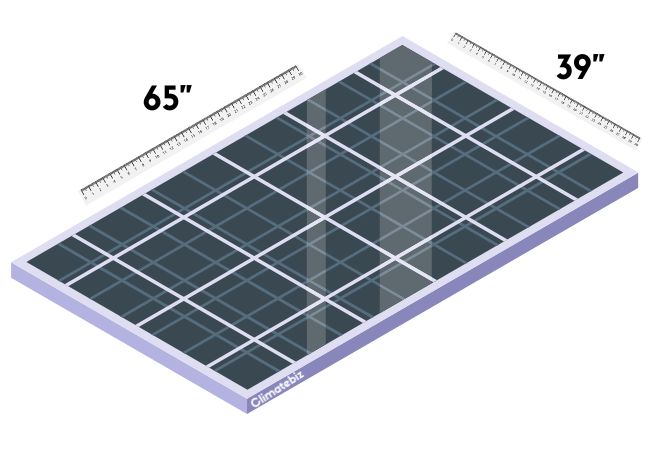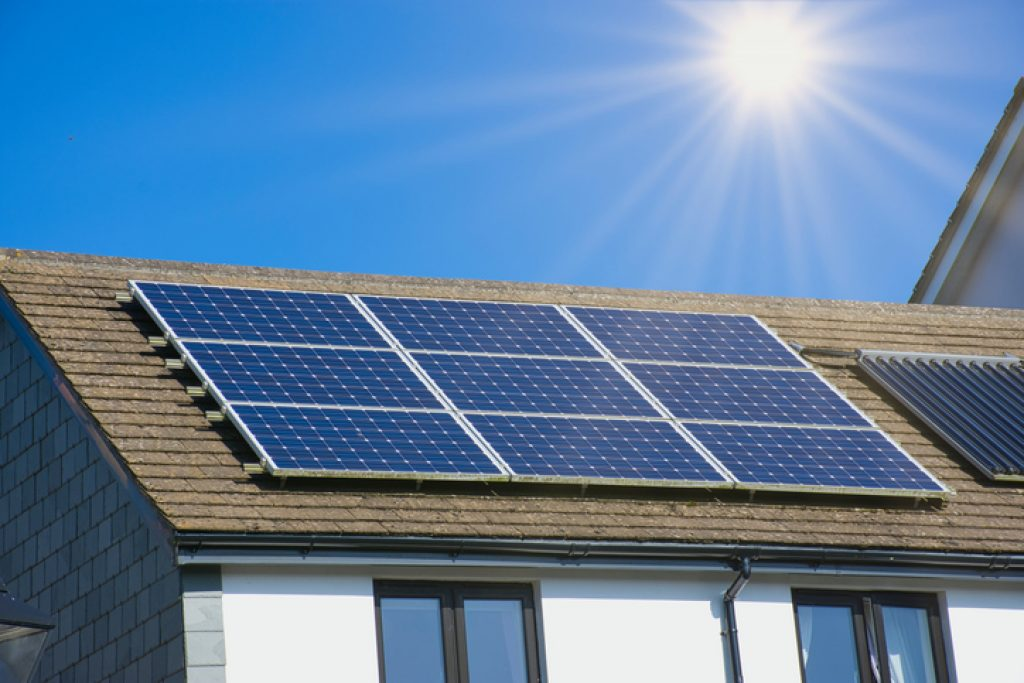# What Size Is A 250-Watt Solar Panel?

Over the past two decades, advancements in solar technology have allowed manufacturers to cater to homeowners’ needs by providing them with panels of various sizes. One such solar panel size that is still in use is the 250-watt module.

Since 250-watt solar panels are relatively affordable, small, and easy to find, they are ideal for residential rooftop installations. As such, knowing the average size of a 250-watt solar panel can really come in handy.

With this information, you can determine the number of 250-watt solar panels you can fit in your desired space, ultimately determining how much energy your system will generate.

In this article, we provide you with the average dimensions of a 250W solar panel. Additionally, we cover the number of 250-watt solar panels you require and how much energy they can produce.

Foreword
Climatebiz experts design, research, fact-check & edit all work meticulously.

### Affiliate Disclaimer

Climatebiz is reader-supported. We may earn an affiliate commission when you buy through links on our site.

## What Size Is A 250-Watt Solar Panel?

The standard size of a 250-watt solar panel is approximately 17.5 ft2 (1.62 m2); its dimensions are 65 x 39 inches (about 1651 x 991 mm).The standard size of solar panels for residential applications (they usually range from 250W to 360W). Source: Climatebiz

However, these numbers can vary slightly depending on the manufacturer, model, and technology used.

We’ve prepared a table that shows the dimensions of five different 250 W solar panels from different brands:

Though the dimensions of these 250-Watt solar panels differ from brand to brand, they don’t deviate much from the standard size previously mentioned.

Related Reading: Solar Panel Dimensions Chart

## How Many 250 Watt Solar Panels Do I Need?

The number of 250- watt solar panels you’ll need depends on:

• The amount of electricity you wish to generate (based on your average energy consumption);
• Efficiency losses due to your home’s location, roof angles, available sunlight, etc.Nine 60-cell solar modules mounted on the roof of a house on a sunny day. Source: smart-energy.com

So, to determine the number of 250-watt solar panels you require, you’ll need to estimate your energy demand, account for efficiency losses, and determine the number of panels (of this size) you can fit in the space available.

There are several ways to estimate your energy demand, but the easiest is simply checking your monthly electric bill — it will tell you the amount of kWh you consume per month.

However, energy consumption changes throughout the year, so you should consider your average annual energy consumption. To do this, add the kilowatt-hours consumed each month of the previous year and divide this number by 12.

According to the U.S. Energy Information Administration, the average annual electricity consumption for a U.S. residential utility customer is around 10,715 kilowatt-hours (kWh), approximately 30 kWh per day.

### Determining The Number Of Solar Panels You Require

If you want your solar system to supply all the energy you consume, you must install an array that can generate the same amount of energy you consume daily.

Example: Let’s consider an energy consumption of 25 kWh and 5 peak sun hours:

First, deduct the efficiency losses (about 20%); this means a 250W solar panel will generate:

250 W – 20% (due to efficiency losses) = 200 W

Now, divide the daily energy consumption by the hours of direct sunlight:

25.000 Wh / 5 hours = 5000 W

Finally, divide this number by the power that one 250W solar panel can generate (after deducting efficiency losses):

5000 W / 200 W = 25 solar panels

### Checking If You Can Fit That Many Panels On Your Roof

You don’t really need an elaborate solar panel square footage calculator to determine the number of panels a roof can support (unless your roof has multiple faces or odd shapes).

There is an easy way to calculate the usable area of your roof:

#### 1) Calculate The Square Footage:

You first need to calculate the square footage of your roof using the length and width dimensions.

#### 2) Account For Setback:

Then, you need to account for the “setback,” which is the free space between the solar array’s edge and the roof’s edge. Doing this establishes an unobstructed pathway around your rooftop in case responders (like firefighters) need to access your home in an emergency.

The minimum solar panel setback varies from state to state, but usually, it’s about 25% of your roof’s space. Therefore, you need to multiply the square footage of your roof by 0.75 to account for the required solar setback.

Example: Let’s say the area of your roof that gets the most sunlight during the day is around 700 ft2; your roofs usable area would be:

Square footage x 0.75 = 700 ft2 x 0.75 = 525 ft2

#### 3) Divide The Usable Roof Area By The Standard Size Of A 250 Watt Solar Panel:

Now that you’ve calculated the usable area of your roof, you need to divide it by 17.5 ft2, the average square footage of the standard 250-watt solar panel size.

The resulting number is the maximum number of 250-watt solar panels you can fit on your home’s roof.

Example: If the usable area is 525 ft2, then:

Usable area / standard size of solar panel = 525 ft2 / 17.5 ft2 = 30

Therefore, you could fit thirty 250-watt solar panels on your roof.

If this is enough to generate the energy you need, great! If not, you’ll need more powerful solar panels (300 W, 350W, or even 400W).

Usually, thirty 250-watt solar modules can generate more than enough energy to power an average U.S. home.

## How Much Energy Can A 250-Watt Solar Panel Produce?

On average, a 250 W solar panel can produce approximately 1 kWh of energy per day (considering 4 peak sun hours and 80% efficiency).

However, this number can vary significantly. Why? Because the amount of energy produced by solar panels depends on multiple factors, such as:

• Location
• Orientation (is the panel facing north, east, west, or south?)
• Tilt/angle (relative to the ground/roof)
• Peak sun hours
• Time of year/season
• Ambient conditions (temperature, humidity, etc.)
• Type of solar panel (monocrystalline, polycrystalline, thin-film)
• Size of solar cell
• Power rating
• Panel efficiency

However, we need to clarify several things:

Solar panels are rated based on how much power they can generate. Let’s recap the difference between power and energy before discussing the energy output of a 250-watt solar panel.

### Energy

In short, energy is the ability to do work. It has various forms: thermal, chemical, nuclear, mechanical, electrical, etc.

Electrical energy results from the movement of electrically charged particles, like electrons.

The unit for electrical energy is Watt-hours (Wh). In other words, energy expresses the power used/generated over a period of time:

Energy (Wh) = power (W) x time (h)

### Power

In physics, power is the amount of energy transferred or converted per unit of time. In other words, it is the rate at which work occurs.

Power (W) = energy (Wh) / time (h)

It can also be calculated as the product of amperage and voltage:

Power (W) = amperage (A) x voltage (V)

The unit for power is watts (W).

Now that we’ve covered how energy and power correlate, we can better understand solar panel energy output.

### Solar Panel Energy Output

A solar panel consists of several solar cells wired in series. Each cell produces a specific voltage and current (depending on its size).

The power rating of a solar panel expresses the maximum power the panel can produce (in ideal conditions). It is usually tested under “Standard Test Conditions” or STC.

This doesn’t mean the panel will produce 250W of power at all times. It means that it can produce a maximum of 250W under ideal conditions hence why it’s so tricky to calculate the energy output of a solar panel.

There are, however, a few ways to estimate solar energy production. The best way is to use an online energy production calculator, like the PVWatts calculator developed by researchers at NREL (National Renewable Energy Laboratory).

Another way is to use the following formula:

Energy produced per day = Average peak sun hours × solar panel wattage x 80% efficiency

Please note: the 80% used in the formula accounts for all the efficiency losses that result from the previously mentioned factors.

Example: For a 250 W solar panel, considering 4.5 peak sun hours:

Energy produced per day = 4.5 hours × 250 W x 0.8 = 900 Wh = 0.9 kWh

In summary, the energy produced by a 250-watt solar panel varies depending on how many hours of direct sunlight hits the panel.

In addition, several other factors result in efficiency losses. Therefore, a 250W solar panel doesn’t produce 250 watt-hours of energy per hour; the amount is always a bit less.

## Final Thoughts

The standard size of a 250-watt solar panel is around 17.5 ft2 (1.62 m2), and dimensions are usually 65 x 39 inches (approximately 1651 x 991 mm).

Solar panels with a maximum power output of 250 watts are ideal for residential installations. Their relatively small size makes them easy to install and fit on most roofs. They are also affordable and easy to find.

You must consider several factors to build the optimum solar power system for your needs.

For instance, your average energy consumption, how much usable roof area you have available for the solar array, the location of your home, the angle of your roof, the solar irradiance in your part of the country, the efficiency of the solar panels you’re using, and a variety of other factors.Ana Lejtman

Ana is a Research Chemist with a strong background in Environmental chemistry. She's deeply interested in how chemistry can be applied to the development of green technologies. Her passion for sustainable chemistry and the environment led her to join the Climatebiz team, writing informative articles on renewable energy/green technologies related topics.